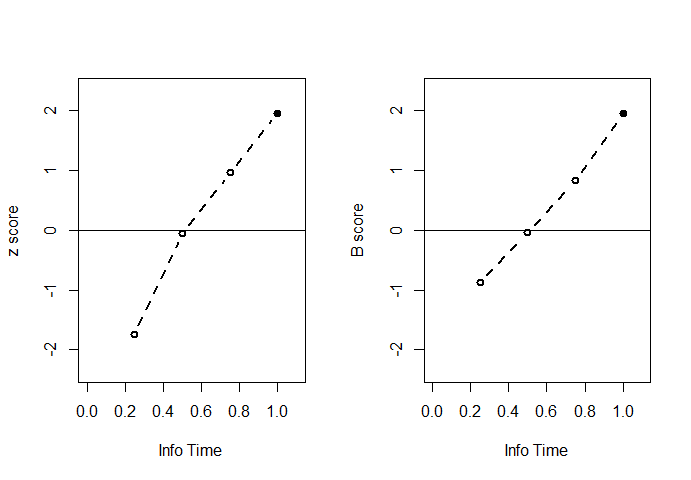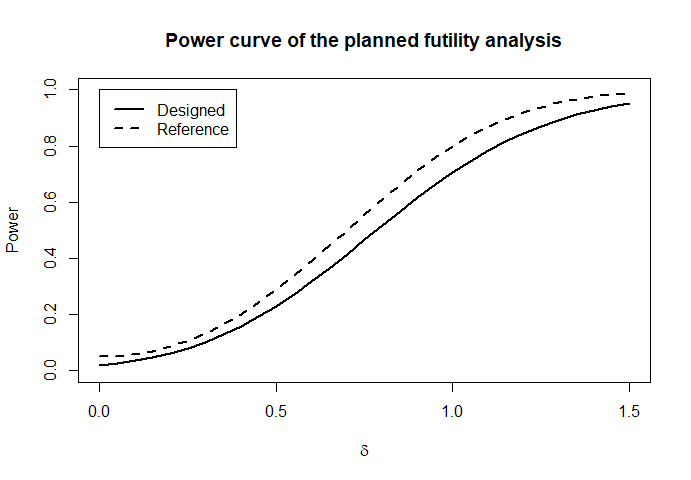# Design of nonbinding futility analysis in clinical trials

## INTRODUCTION

This vignette demonstrates the use of the grpseq package in the design of nonbinding futility analysis in clinical trials (Gallo, Mao, and Shih, 2014, Journal of Biopharmaceutical Statistics).

### Basics of group sequential trials

Suppose a trial aims to enroll $$n$$ patients over time. As patients accrue, one may use the partial cohort to gauge the prospect of achieving positive result on the full cohort. Should the interim results be exceptionally promising or unpromising, one may be obliged by ethical and financial reasons to terminate the trial early, for efficacy or futility, respectively.

#### B-values

Superimposing early termination rules on a trial planned for a single analysis will generally alter its type I error rate and power. To control the operating characteristics of the sequential tests, we need to evaluate not only the distribution of the final test statistic but also its joint distribution with the interim ones. Formulating these statistics in terms of the “B-value” simplifies this evaluation (Lan and Wittes, 1988).

Consider testing $$H_0: \theta=0$$ against $$H_A: \theta>0$$, where $$\theta$$ is some (standardized) metric of effect size, such as the mean difference between treatment and control (in units of standard deviation). Given a sample of size $$n$$, let the test statistic be $$Z_n=\sqrt n T_n$$, where $$T_n$$ is an estimator for $$\theta$$, e.g., a standardized sample average. This typically leads to $$Z_n\sim N(\sqrt n\theta, 1)$$, which implies that $$Z_n\sim N(0, 1)$$ under $$H_0$$. In that sense, $$h=\sqrt n\theta$$ can be viewed as the “noncentrality parameter” of the test statistic, or “local alternative” under $$H_A$$.

Suppose that we plan to perform $$K-1$$ interim analyses when the cohort size reaches $$n_1<\cdots<n_{K-1}$$ (where $$n_{K-1}<n$$). Along with the final analysis at $$n_K:=n$$, the operating characteristics are determined by the joint distribution of $$(Z_{n_1},\ldots, Z_{n_{K}})^{\rm T}$$ under $$H_0$$ and $$H_A$$. To derive it, we re-scale the $$Z_{n_k}$$ into the $$B$$-statistics by $$B(t_k)=\sqrt{t_k}Z_{n_k}$$, where $$t_k=n_k/n$$ is the “information time” at the $$k$$th look (sample size is proportional to statistical information). Under large samples, the test statistic usually behaves like a sample average, that is, $$Z_{n_k}\approx\sqrt{n_k}\theta+n_k^{-1/2}\sum_{i=1}^{n_k}X_i$$ for some mean-zero $$X_i$$ with $$E(X_i^2)=1$$ and $$E(X_iX_j)=0$$ for $$i\neq j$$. This means that $$B(t_k)\approx ht_k+n^{-1/2}\sum_{i=1}^{n_k}X_i$$, which clearly has independent increments in the sense that $$B(t)\perp \{B(s)-B(t)\}$$ for $$s>t$$. In fact, by the multivariate central limit theorem, $\begin{equation}\tag{1} \left(\begin{array}{c}B(t_1)\\B(t_2)\\\vdots\\B(t_{K-1})\\B(1)\end{array}\right) \sim N \left\{ h\left(\begin{array}{ccccc}t_1\\t_2\\\vdots\\t_{K-1}\\1\end{array}\right), \left(\begin{array}{c}t_1& t_1&\ldots& t_1&t_1 \\ t_1& t_2&\ldots& t_2&t_2\\ \vdots&\vdots&\vdots&\vdots&\vdots\\ t_1& t_2 & \cdots & t_{K-1}& t_{K-1}\\ t_1& t_2 & \cdots & t_{K-1}& 1 \end{array}\right) \right\}. \end{equation}$ Therefore, the left hand side can be viewed discrete evaluations of a continuous-time Brownian motion $$B(t)$$ satisfying $${\rm cov}\{B(t),B(s)\}=\min(t, s)$$ and with drift term $$E\{B(t)\}=ht$$.

#### Conditional power and predictive power

For a one-off analysis of the full cohort, we reject $$H_0:\theta=0$$ if $$|B(1)|>z_{1-\alpha/2}$$, where $$\alpha$$ is the type I error and $$z_{1-\alpha/2}=\Phi^{-1}(1-\alpha/2)$$, and $$\Phi(\cdot)$$ is the cumulative distribution function of the standard normal distribution. At $$\theta>0$$, or rather, $$h=\sqrt n\theta>0$$, the power of the test is approximately $$P\{B(1)> z_{1-\alpha/2}\mid h\}=\Phi(h-z_{1-\alpha/2})$$ (since $$P\{B(1)< -z_{1-\alpha/2}\mid h\}\approx 0$$ for any moderately sized $$h>0$$) so that $$h=z_{1-\alpha/2}+z_{1-\beta}$$ if the target power is $$1-\beta$$.

At information time $$t_k$$, we have that $$B(t)\mid \{B(t)=b\}\sim N\{b+h(1-t), 1-t\}$$ by (1), so the conditional power (CP) is $\begin{equation}\tag{2} P\{B(1) > z_{1-\alpha/2} \mid B(t)=b; h\}=\Phi\left(\frac{b+h(1-t)-z_{1-\alpha/2}}{\sqrt{1-t}}\right). \end{equation}$ Replacing $$h$$ with $$z_{1-\alpha/2}+z_{1-\beta}$$ establishes the conditional power as a function of the target power.

The left hand side of (2) is evaluated under a pre-specified local alternative, which may no longer be tenable in light of the data accrued by time $$t$$. A more data-adaptive approach substitutes $$h$$ for its estimator $$\hat h_t=t^{-1}B(t)$$ (since $$E\{B(t)\}=ht$$). That leads to the conditional power under the current estimate (CPd): $\begin{equation}\tag{3} P\{B(1) > z_{1-\alpha/2} \mid B(t)=b; \hat h_t\}=\Phi\left(\frac{t^{-1}b-z_{1-\alpha/2}}{\sqrt{1-t}}\right). \end{equation}$ This nonetheless has the limitation of treating $$\hat h_t$$ as if it were fixed. To account for its randomness, we can average the left hand side of (2) over the its posterior distribution given $$B(t)$$. Because $$B(t)\mid h \sim N(ht, t)$$, we easily obtain that $$h\mid \{B(t)=b\}\sim N(t^{-1}b, t^{-1})$$ under an improper flat prior for $$h$$. This leads to the predictive power (PP) $\begin{equation}\tag{4} P\{B(1) > z_{1-\alpha/2} \mid B(t)=b\}=\Phi\left(\frac{b-tz_{1-\alpha/2}}{\sqrt{t(1-t)}}\right). \end{equation}$

### Nonbinding futility analysis

In a futility design, we stop the trial to accept $$H_0$$ when the CP, CPd, or PP drops below a certain threshold, indicating slim chance of rejecting it eventually. The corresponding bound for the B-value can be obtained by solving (2), (3), or (4) for $$b$$ (and thus the z-value by $$z=b/\sqrt t$$) at each information time. Obviously, adding futility rules increases the change of accepting $$H_0$$, thereby shrinking both the type I error and power. To “use up” the nominal type I error so as to recoup power, one can lower the critical value $$z_{1-\alpha}$$ to make rejection easier (and perhaps re-evaluating (2)–(4) iteratively to get the precise amount of decrease). But this would make the futility rules binding — failing to stop when the boundaries are crossed leads to inflated type I error. It is thus more popular to maintain the critical value and either to inflate the sample size to recoup power (recall that $$\sqrt n\theta=h=z_{1-\alpha/2}+z_{1-\beta}$$) or to evaluate the power loss and see if it is acceptable. This allows us to treat futility boundaries as nonbinding guidelines rather than rigid rules.

Let $$b_k$$ denote the futility bound for the $$B$$-statistic at $$t_k$$ $$(k=1,\ldots, K-1)$$. Then the power lost by enforcing this bound is the probability of accepting $$H_0$$ at $$t_k$$ while the final analysis could have rejected it, namely, $\psi(t_k)=P\{B(t_1)\geq b_1,\ldots, B(t_{k-1})\geq b_{k-1}, B(t_k)<b_k, B(1)>z_{1-\alpha/2} \mid h\},$ which can be evaluated based on the multivariate normal distribution in (1). The total power loss is thus $$\sum_{k=1}^{K-1}\psi(t_k)$$. Alternatively, we can assess the “beta” (type II error) spent at each look by evaluating $$P\{\mbox{accepting }H_0\mbox{ at }t_k\mid h\}$$ along similar lines.

Another important metric for the quality of the futility boundaries is the expected sample size under $$H_0$$. The smaller the sample size, the less costly (in both financial or ethical terms) the trial. By similar logic to the calculation of power loss and beta spent, we can calculate the expected sample size as a fraction of the original sample size by $$\sum_{k=1}^{K} t_kP\{\mbox{stopping at }t_k\mid h=0\}$$. With sample size inflation, simply multiply it by the inflation factor.

## BASIC SYNTAX AND EXAMPLE

The main function for planning nonbinding futility analysis is fut(). Its basic syntax is of the form

obj <- fut(alpha, beta, t, gamma, side = 2, si = 0, scale = "CP")

In the above, alpha and beta are the target type I and II errors, respectively, t is a vector of information times for the futility looks, gamma is the corresponding vector of specified CP, CPd, or PP when scale = "CP", "CPd", and "PP", respectively, side specifies whether the test is one- or two-sided, and si = 1 if one wants to inflate sample size to recoup power (si = 0 to leave it as it is). Summary information of the planned analysis can be printed out by

summary(obj)

The printout includes the calculated $$B$$- and $$z$$-values, beta spent, and power loss (if si=0) at each of the specified information times along with the expected sample size under $$H_0$$ and the sample size inflation factor (if si=1). Using the plot() and powerplot() functions on obj plots the futility bounds (in terms B- or z-values) and the power curve of the planned analysis as a function of the local effect size (in units of the hypothesized effect size), respectively.

For example, consider a two-sided test with type I error 0.05 and power 0.8, and suppose that we want to impose three futility looks at information times 0.25, 0.50, and 0.75. We want to set the futility bounds to correspond to 20% PP. That is, we drop the trial for futility if the PP dips below 20% when the patient cohort reaches a quarter, half, and three quarters of the target size. First, we take the sample size inflation approach to avoid losing power:

## load the package
library(grpseq)
## two-sided level 0.05 test with 80% power;
## evenly spaced three futility looks with predictive power 20%;
## inflate sample size to recoup power.
obj1 <- fut(alpha=0.05,beta=0.2,t=(1:3)/4,gamma=0.2*rep(1,3),side=2,scale="PP",si=1)
obj1
#>
#> Call:
#> fut(alpha = 0.05, beta = 0.2, t = (1:3)/4, gamma = 0.2 * rep(1,
#>     3), side = 2, si = 1, scale = "PP")
#>
#> Sample Size inflation factor: 1.156737

This tells us that we need to inflate the sample size by 1.16 to restore the 80% power. Now, consider the same set-up without sample size inflation.

## do the same thing without sample size inflation
obj2 <- fut(alpha=0.05,beta=0.2,t=(1:3)/4,gamma=0.2*rep(1,3),side=2,scale="PP",si=0)
obj2
#>
#> Call:
#> fut(alpha = 0.05, beta = 0.2, t = (1:3)/4, gamma = 0.2 * rep(1,
#>     3), side = 2, si = 0, scale = "PP")
#>
#> Power loss due to futility rules: 0.09388177 (planned power: 0.8).
## print the summary results
summary(obj2)
#>
#> Call:
#> fut(alpha = 0.05, beta = 0.2, t = (1:3)/4, gamma = 0.2 * rep(1,
#>     3), side = 2, si = 0, scale = "PP")
#>
#> Sample Size inflation factor: 1
#> Nominal type I error = 0.05, Power loss = 0.0939 (Planned power: 0.8),
#> Side = 2
#>
#>            Interim 1 Interim 2 Interim 3 Final
#> Info Time     0.2500    0.5000    0.7500  1.00
#> PP            0.2000    0.2000    0.2000    NA
#> B-Value       0.1256    0.5592    1.1055  1.96
#> Z-value       0.2511    0.7908    1.2766  1.96
#> Beta spent    0.1251    0.0568    0.0421  0.07
#> Power Loss    0.0638    0.0208    0.0093    NA
#>
#>
#> Sample size distribution under H_0:
#> (as a ratio to that of the reference test)
#> Expected sample size: 0.4124
#>
#>             Interim 1 Interim 2 Interim 3 Final
#> Sample Size    0.2500    0.5000    0.7500 1.000
#> Probability    0.5991    0.2253    0.1026 0.073

We can see that the imposed futility looks take away 9.4% power from the target 80%. On the other hand, they cut the expected sample size by 1-41.2%=58.8% under $$H_0$$. The listed boundaries for the B- and z-values can be plotted by

oldpar <- par(mfrow = par("mfrow"))
par(mfrow=c(1,2))
## plot the futility boundaries by z-value
plot(obj2,scale='z',lwd=2,main="")
## plot the futility boundaries by B-value
plot(obj2,scale='b',lwd=2,main="")par(oldpar)

Finally, we plot the power curve of the planned futility analysis with reference to that of the original test.

## plot the power curve as a function of the (local)
## effect size in units of the hypothesized effect size
## ref=TRUE requests the power curve for the original one-time analysis
powerplot(obj2,lwd=2, ref=TRUE)The difference between the solid and dashed lines at $$\delta=1$$ reflects the 9.4% power loss due to the futility looks.